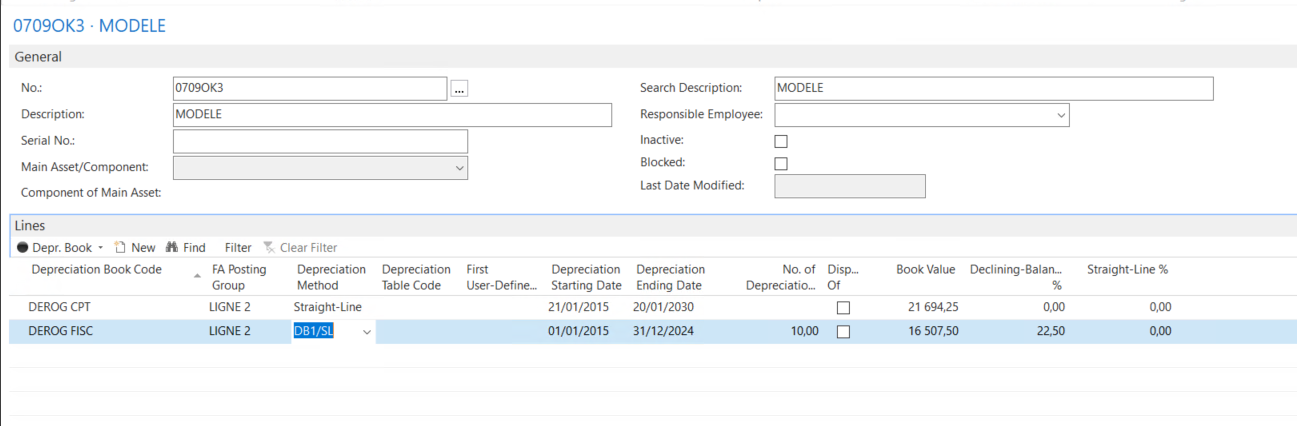#### Howdy, Stranger!

It looks like you're new here. Sign in or register to get started.

# Calculate derogatory depreciation quarterly instead of yearly ?

Hello everyone,

One of our client wants to calculate his derogatory depreciations quarterly instead of yearly, but the calculation doesn't seem to work.To be a bit more specific, i have here an example with a linear depreciation calculated over 15 years and a derogatory depreciation calculated over 10.
As long as i use the Calculate Depreciation method over a whole year, the calculation works fine but if i try a calculation over 3 months the results of the derogaroty depreciation are wrong (the linear works fine).
Is there something i'm doing wrong ? Should i use a different depreciation method ?
Also adding that we're based in France since it can influence the calculations.

Any help will be highly appreciated !